# Lie algebra, solvable

(diff) ← Older revision | Latest revision (diff) | Newer revision → (diff)

A Lie algebraover a fieldsatisfying one of the following equivalent conditions:

1) the terms of the derived seriesofare equal to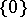for sufficiently large;

2) there is a finite decreasing chain of idealsofsuch that,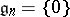and(that is, the Lie algebras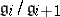are Abelian for all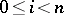);

3) there is a finite decreasing chain of subalgebras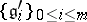such that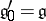,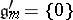,is an ideal of, and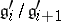is a one-dimensional (Abelian) Lie algebra for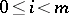.

A nilpotent Lie algebra (cf. Lie algebra, nilpotent) is solvable. Ifis a complete flag in a finite-dimensional vector spaceover, thenis a solvable subalgebra of the Lie algebra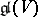of all linear transformations of. If one chooses a basis incompatible with, then with respect to that basis, elements of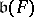are represented by upper triangular matrices; the resulting solvable linear Lie algebra is denoted by, where.

The class of solvable Lie algebras is closed with respect to transition to a subalgebra, a quotient algebra or an extension. In particular, any subalgebra of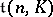is solvable. If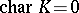andis an algebraically closed field, then any finite-dimensional solvable Lie algebra is isomorphic to a subalgebra offor some. One of the main properties of solvable Lie algebras is expressed in Lie's theorem: Letbe a solvable Lie algebra over an algebraically closed field of characteristicand letbe a finite-dimensional linear representation of it. Then inthere is a complete flagsuch that. In particular, ifis irreducible, then. Ideals ofcan be chosen so as to form a complete flag, that is, so that.

A finite-dimensional Lie algebraover a field of characteristicis solvable if and only if the algebra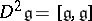is nilpotent. Another criterion for solvability (Cartan's criterion) is:is solvable if and only if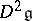is orthogonal to the whole ofwith respect to the Killing form (or any bilinear form associated with a faithful finite-dimensional representation of).

Solvable Lie algebras were first considered by S. Lie in connection with the study of solvable Lie transformation groups. The study of solvable Lie algebras acquired great significance after the introduction of the concept of the radical (that is, the largest solvable ideal) of an arbitrary finite-dimensional Lie algebra, and it was proved that in the casethe algebrais the semi-direct sum of its radical and a maximal semi-simple subalgebra (see Levi–Mal'tsev decomposition). This made it possible to reduce the problem of classifying arbitrary Lie algebras to the enumeration of semi-simple (which for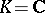had already been done by W. Killing) and solvable Lie algebras. The classification of solvable Lie algebras (foror) has been carried out only in dimensions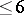.

Ifis a solvable algebraic subalgebra (cf. Algebraic algebra) of, whereis a finite-dimensional space over a fieldof characteristic, thensplits into the semi-direct product of the nilpotent ideal formed by all nilpotent transformations ofand the Abelian subalgebra consisting of the semi-simple transformations . In general, any split solvable Lie algebra, that is, a finite-dimensional solvable Lie algebra overevery elementof which splits into a sum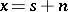, where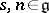,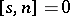,is semi-simple, andis nilpotent, has a similar structure . To every finite-dimensional solvable Lie algebra overthere uniquely corresponds a minimal split solvable Lie algebra containing it (the Mal'tsev decomposition). The problem of classifying solvable Lie algebras that have a given Mal'tsev decomposition has been solved . Thus, the problem of classifying solvable Lie algebras reduces, in a certain sense, to the study of nilpotent Lie algebras.

Apart from the radical, in an arbitrary finite-dimensional Lie algebraone can distinguish maximal solvable subalgebras. Ifis an algebraically closed field of characteristic, then all such subalgebras of(they are called Borel subalgebras) are conjugate. For example,is a Borel subalgebra of the Lie algebra of all matrices of order. Ifis not algebraically closed or ifis finite, then Lie's theorem is false, in general. However, it can be extended to the case whenis perfect and contains the characteristic roots of all the characteristic polynomialsof the adjoint transformations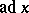,. If this condition is satisfied for the adjoint representation of a solvable Lie algebra(cf. Adjoint representation of a Lie group), thenis said to be triangular. Many properties of solvable Lie algebras over an algebraically closed field carry over to triangular Lie algebras. In particular, if, then all maximal triangular subalgebras of an arbitrary finite-dimensional Lie algebra are conjugate (see , ). Maximal triangular subalgebras are used in the study of semi-simple Lie algebras over an algebraically non-closed field as a good analogue of Borel subalgebras. They also play a fundamental role in the description of the connected uniform subgroups (cf. Uniform subgroup) of Lie groups .

How to Cite This Entry:
Lie algebra, solvable. Encyclopedia of Mathematics. URL: http://encyclopediaofmath.org/index.php?title=Lie_algebra,_solvable&oldid=17061
This article was adapted from an original article by V.V. Gorbatsevich (originator), which appeared in Encyclopedia of Mathematics - ISBN 1402006098. See original article